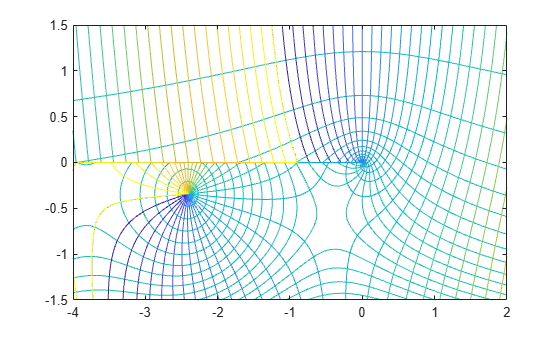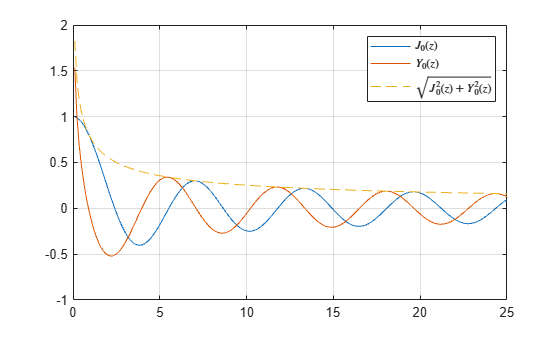# besselh

Bessel function of third kind (Hankel function)

## Syntax

H = besselh(nu,Z)
H = besselh(nu,K,Z)
H = besselh(nu,K,Z,scale)

## Description

example

H = besselh(nu,Z) computes the Hankel function of the first kind ${H}_{\nu }^{\text{\hspace{0.17em}}\left(1\right)}\left(z\right)={J}_{\nu }\left(z\right)+i{Y}_{\nu }\left(z\right)$ for each element in array Z.

example

H = besselh(nu,K,Z) computes the Hankel function of the first or second kind ${H}_{\nu }{}^{\left(K\right)}\left(z\right)$, where K is 1 or 2, for each element of array Z.

example

H = besselh(nu,K,Z,scale) specifies whether to scale the Hankel function to avoid overflow or loss of accuracy. If scale is 1, then Hankel functions of the first kind ${H}_{\nu }^{\left(1\right)}\left(z\right)$ are scaled by ${e}^{-iZ}$, and Hankel functions of the second kind ${H}_{\nu }^{\left(2\right)}\left(z\right)$ are scaled by ${e}^{+iZ}$.

## Examples

collapse all

Generate the contour plots of the modulus and phase of the Hankel function ${H}_{0}^{\left(1\right)}\left(z\right)$ .

Create a grid of values for the domain.

[X,Y] = meshgrid(-4:0.002:2,-1.5:0.002:1.5);

Calculate the Hankel function over this domain and generate the modulus contour plot.

H = besselh(0,X+1i*Y); contour(X,Y,abs(H),0:0.2:3.2) hold onIn the same figure, add the contour plot of the phase.

contour(X,Y,rad2deg(angle(H)),-180:10:180) hold offPlot the real and imaginary parts of the Hankel function of the second kind and examine their asymptotic behavior.

Calculate the Hankel function of the second kind ${\mathit{H}}_{0}^{\left(2\right)}\left(\mathit{z}\right)={\mathit{J}}_{0}\left(\mathit{z}\right)-\mathit{i}{\mathit{Y}}_{0}\left(\mathit{z}\right)$ in the interval $\left[0.1,\text{\hspace{0.17em}}25\right]$.

k = 2; nu = 0; z = linspace(0.1,25,200); H = besselh(nu,k,z);

Plot the real and imaginary parts of the function. In the same figure, plot the linear combination $\sqrt{{\mathit{J}}_{0}^{2}\left(\mathit{z}\right)+{\mathit{Y}}_{0}^{2}\left(\mathit{z}\right)}$, which reveals the asymptotic behavior of the magnitudes of the real and imaginary parts.

plot(z,real(H),z,imag(H)) grid on hold on M = sqrt(real(H).^2 + imag(H).^2); plot(z,M,'--') legend('$J_0(z)$', '$Y_0(z)$', '$\sqrt{J_0^2 (z) + Y_0^2 (z)}$','interpreter','latex')Calculate the exponentially scaled Hankel function ${\mathit{H}}_{1}^{\left(2\right)}\left(\mathit{z}\right){\text{\hspace{0.17em}}\cdot \text{\hspace{0.17em}}\mathit{e}}^{\mathrm{iz}}$ on the complex plane and compare it to the unscaled function.

Calculate the unscaled Hankel function of the second order on the complex plane. When z has a large positive imaginary part, the value of the function quickly diverges. This phenomenon limits the range of computable values.

k = 2; nu = 1; x = -5:0.4:15; y = x'; z = x + 1i*y; scaled = 1; H = besselh(nu,k,z); surf(x,y,imag(H)) xlabel('real(z)') ylabel('imag(z)')Now, calculate ${\mathit{H}}_{1}^{\left(2\right)}\left(\mathit{z}\right){\text{\hspace{0.17em}}\cdot \text{\hspace{0.17em}}\mathit{e}}^{\mathrm{iz}}$ on the complex plane and compare it to the unscaled function. The scaled function increases the range of computable values by avoiding overflow and loss of accuracy when z has a large positive imaginary part.

Hs = besselh(nu,k,z,scaled); surf(x,y,imag(Hs)) xlabel('real(z)') ylabel('imag(z)')## Input Arguments

collapse all

Equation order, specified as a scalar, vector, matrix, or multidimensional array. nu specifies the order of the Hankel function. nu and Z must be the same size, or one of them can be scalar.

Example: besselh(3,Z)

Data Types: single | double

Kind of Hankel function, specified as 1 or 2.

• If K = 1, then besselh computes the Hankel function of the first kind ${H}_{\nu }^{\text{\hspace{0.17em}}\left(1\right)}\left(z\right)={J}_{\nu }\left(z\right)+i{Y}_{\nu }\left(z\right)$.

• If K = 2, then besselh computes the Hankel function of the second kind ${H}_{\nu }^{\left(2\right)}\left(z\right)={J}_{\nu }\left(z\right)-i{Y}_{\nu }\left(z\right)$.

Example: besselh(nu,2,Z)

Functional domain, specified as a scalar, vector, matrix, or multidimensional array. nu and Z must be the same size, or one of them can be scalar.

Example: besselh(nu,[1-1i 1+0i 1+1i])

Data Types: single | double
Complex Number Support: Yes

Toggle to scale function, specified as one of these values:

• 0 (default) — No scaling

• 1 — Scale the output of besselh, depending on the value of K:

• If K = 1, then scale the Hankel function of the first kind ${H}_{\nu }^{\left(1\right)}\left(z\right)$ by ${e}^{-iZ}$.

• If K = 2, then scale Hankel function of the second kind ${H}_{\nu }^{\left(2\right)}\left(z\right)$ by ${e}^{+iZ}$.

On the complex plane, ${H}_{\nu }^{\left(1\right)}\left(z\right)$ overflows when imag(Z) is large and negative. Similarly, ${H}_{\nu }^{\left(2\right)}\left(z\right)$ overflows when imag(Z) is large and positive. Exponentially scaling the output of besselh is useful in these two cases since the function otherwise quickly loses accuracy or overflows the limits of double precision.

Example: besselh(nu,K,Z,1)

collapse all

### Hankel Functions and Bessel’s Equation

This differential equation, where ν is a real constant, is called Bessel's equation:

${z}^{2}\frac{{d}^{2}y}{d{z}^{2}}+z\frac{dy}{dz}+\left({z}^{2}-{\nu }^{2}\right)y=0.$

Its solutions are known as Bessel functions.

The Bessel functions of the first kind, denoted Jν(z) and Jν(z), form a fundamental set of solutions of Bessel's equation for noninteger ν. The Bessel functions of the second kind, denoted Yν(z), form a second solution of Bessel's equation—linearly independent of Jν(z)—defined by

${Y}_{\nu }\left(z\right)=\frac{{J}_{\nu }\left(z\right)\mathrm{cos}\left(\nu \pi \right)-{J}_{-\nu }\left(z\right)}{\mathrm{sin}\left(\nu \pi \right)}.$

The Bessel functions of the third kind, also called Hankel functions of the first and second kind, are defined by linear combinations of the Bessel functions, where Jν(z) is besselj, and Yν(z) is bessely:

$\begin{array}{l}{H}_{\nu }^{\left(1\right)}\left(z\right)={J}_{\nu }\left(z\right)+i{Y}_{\nu }\left(z\right)\\ {H}_{\nu }^{\left(2\right)}\left(z\right)={J}_{\nu }\left(z\right)-i{Y}_{\nu }\left(z\right).\end{array}$

 Abramowitz, M., and I.A. Stegun. Handbook of Mathematical Functions. National Bureau of Standards, Applied Math. Series #55, Dover Publications, 1965.